# 2013 A-level H2 Mathematics (9740) Paper 1 Question 9 Suggested SolutionsAll solutions here are SUGGESTED. Mr. Teng will hold no liability for any errors. Comments are entirely personal opinions.

(i)
Let P(n) be the prepositionWhen, P(1) is true.

Assume the P(k) is true for someWant to prove that P(k+1) is also true, i.e.,Since P(1) is true, and P(k) is trueP(k+1) is true, by Mathematical Induction, P(n) is true for all(ii)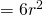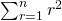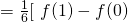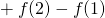(iii)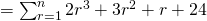Not readable? Change text.